An algebraic expression is a description of certain calculations that have to be done in a certain order. In this chapter, you will be introduced to the language of algebra. You will also learn about expressions that appear to be different but that produce the same results when evaluated. When we evaluate an expression, we choose or are given a value of the variable in the expression. Because now we have an actual value, we can carry out the operations ($+, -, \times, \div)$ in the expression using this value.

## Algebraic language

### Words, diagrams and symbols

1. Complete this table
 Words Flow diagram Expression Multiply a number by two and add six to the answer.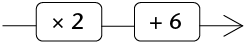$2 \times x + 6$ (a) Add three to a number and then multiply the answer by two. (b)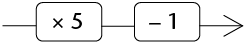(c) $7 + 4 \times x$ (d) $10 - 5 \times x$

An algebraic expression indicates a sequence of calculations that can also be described in words or with a flow diagram.

The flow diagram illustarte the order in which the calculations must be done

In algebraic language the multipliaction sign is usually ommited. So we write $2x$ instead of $2 \times x$

We also write $x \times 2 \text{ as } 2x$

1. Write the following expressions in 'normal' algebraic language:
1. $2 \times a + b$
2. $a2$

### Looking different but yet the same

1. Complete the table by calculating the numerical values of the expressions for the values of $x$. Some answers for $x$ = 1 have been done for you as an example.
 $x$ 1 3 7 10 (a) $2x + 3x$ $2 \times 1 + 3 \times 1$ $2 + 3 = 5$ (b) $5x$ (c) $2x + 3$ (d) $5x^2$ $5 \times (1)^2$ $5 \times 1 = 5$
2. Do the expressions $2x + 3$ and $5x$, in question 1 above, produce different answers or the same answer for:
1. $x = 3$?
2. $x = 10$?
3. Do the expressions $2x + 3$ and $5x$ produce different answers or the same answer for:
1. $x = 3$?
2. $x = 10$?
4. Write down all the algebraic expressions in question 1 that have the same numerical value for the same value(s) of $x$, although they may look different. Justify your answer.

One of the things we do in algebra is to evaluate expressions. When we evaluate an expression we choose or are given a value of the variable in the expression. Because now we have an actual value we can carry out the operations in the expression using this value, as in the examples given in the table.

Algeraic expressions that have the same numarical value for the same value of x but look different are called equivalent expressions.

1. Say whether the following statements are true or false. Explain your answer in each case.
1. The expressions $2x + 3x$ and $5x$ are equivalent.
2. The expressions $2x + 3$ and $5x$ are equivalent.
2. Consider the expressions $3x + 2z + y$ and $6xyz$.

Remember that $6xyz$ is the same as $6 \times x \times y \times z$.

1. What is the value of $3x + 2z + y$ for $x = 4$ , $y = 7$ and $z = 10$?
2. What is the value of $6xyz$ for $x = 4$ , $y = 7$ and $z = 10$?
3. Are the expressions $3x + 2z + y$ and $6xyz$ equivalent? Explain.

To show that the two expressions in question 5(a) are equivalent we write $2x + 3x = 5x$.

We can explain why this is so:

$2x + 3x = (x + x) + (x + x + x) = 5x$

We say the expression $2x + 3x$ simplifies to $5x$.

The term $3x$ is a product. The number 3 is called the coefficient of $x$.

1. In each case below, write down an expression equivalent to the one given.
1. $3x + 3x$
2. $3x + 8x + 2x$
3. $8b + 2b + 2b$
4. $7m + 2m + 10m$
5. $3x^2 + 3x^2$
6. $3x^2 + 8x^2 + 2x^2$
2. What is the coefficient of $x^2$ for the expression equivalent to $3x^2 + 8x^2 + 2x^2$?

In an expression that can be written as a sum, the different parts of the expression are called the terms of the expression. For example, $3x,2z \text{ and } y$ are the terms of the expression $3x + 2z + y$.

An expression can have like terms or unlike terms or both.

Like terms are terms that have the same variable(s) raised to the same power. The terms $2x$ and $3x$ are examples of like terms.

1. Calculate the numerical value of $10x + 2y$ for $x = 3$ and $y = 2$ by completing the empty spaces in the diagram.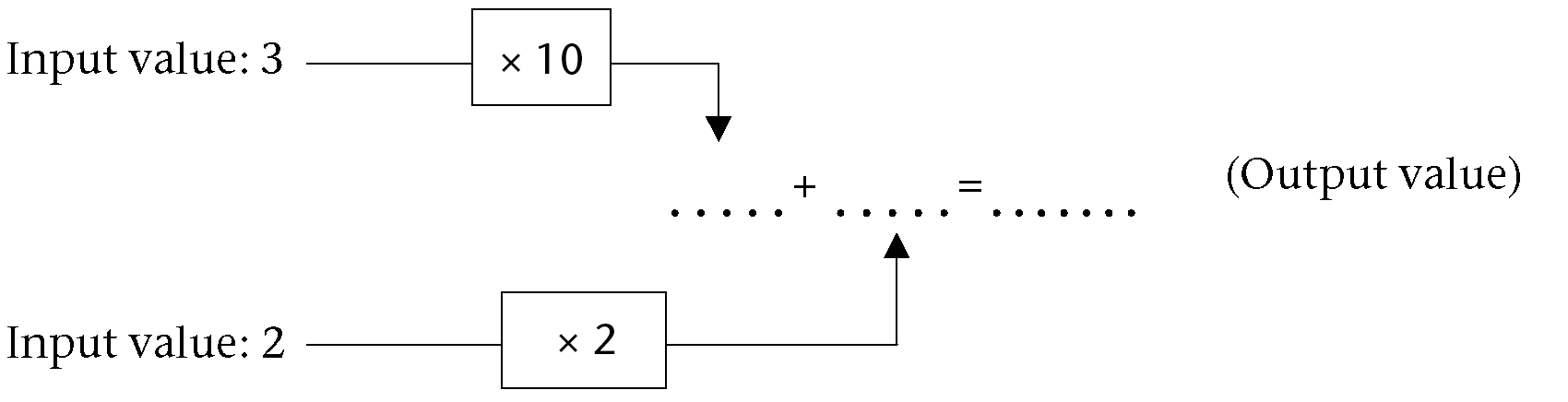2. What is the output value for the expression $12xy$ for $x = 3 \text{ and } y = 2$?
3. Are the expressions $10x +2y$ and $12xy$ equivalent? Explain.
4. Are the terms $10x$ and $2y$ like or unlike terms? Explain.
1. Which of the following algebraic expressions do you think will give the same results?

A. $6x + 4x$

B. $10x$

C. $10x^2$

D. $9x + x$

2. Test the algebraic expressions you have identified for the following values of $x$

$x = 10$

$x = 17$

$x = 54$

3. Are the terms $6x$ and $4x$ like or unlike terms? Explain.
4. Are the terms $10x$ and $10x^2$ like or unlike terms? Explain.
1. Ashraf and Hendrik have a disagreement about whether the terms $7x^2y^3$ and $301y^3x^2$ are like terms or not. Hendrik thinks they are not, because in the first term the $x^2$ comes before the $y^3$ whereas in the second term $y^3$ comes before the $x^2$.

Explain to Hendrik why his argument is not correct.

2. Explain why the terms $5abc, 10acb \text{ and } 15cba$ are like terms.

## Add and subtract like terms

### Rearrange terms and then combine like terms

1. Complete the table by evaluating the expressions for the given values of $x$.
 $x$ 1 2 10 $30x + 80$ $30 \times 1 + 80$ $= 30 + 80 = 110$ $5x + 20$ $30x + 80 + 5x + 20$ $35x + 100$ $135x$
2. Write down all the expressions in the table that are equivalent.
3. Tim thinks that the expressions $135x$ and $35x +100$ are equivalent because for $x = 1$ they both have the same numerical value 135.

Explain to Tim why the two expressions are not equivalent.

We have already come across the commutative and associative properties of operations. We will now use these properties to help us form equivalent algebraic expressions.

Commutative property

The order in which we add or multiply numbers does not change the answer: $a + b = b + a$ and $ab = ba$

Associative property

The way in which we group three or more numbers when adding or multiplying does not change the answer: $(a +b)+c = a + (b + c)$ and $(ab)c = a(bc)$

We can find an equivalent expression by rearranging and combining like terms, as shown below:

$30x + 80 + 5x + 20$

Hence $30x + (80 + 5x) + 20$

Hence $30x + (80 + 5x) + 20$

$= (30x + 5x) + (80 + 20)$

$= 35x + 100$

The terms 80 and 20 are called constants. The numbers 30 and 5 are called coefficients.

Brackets are used in the expression above to show how the like terms have been rearranged.

Like terms are combined to form a single term.

The terms $30x$ and $5x$ are combined to get the new term $35$ and the terms 80 and 20 are combined to form the new term 100. We say that the expression $30x + 80 + 5x + 20$ is simplified to a new expression $35x + 100$.

1. Simplify the following expressions:
1. $13x + 7 + 6x - 2$
2. $21x - 8 + 7x + 15$
3. $8c - 12d + 5c - 7c$
4. $3abc + 4 + 7abc - 6$
5. $12x^2 + 2x - 2x^2 + 8x$
6. $7m^3 + 7m^2 + 9m^3 + 1$

When you are not sure about whether you correctly simplified an expression, it is always advisable to check your work by evaluating the original expression and the simplified expression for some values. This is a very useful habit to have.

When we use a value of the variable in the expression we call that substitution.

1. Make a simpler expression that is equivalent to the given expression. Test your answer for three different values of x, and redo your work until you get it right.
1. $(15x + 7y) + (25x + 3 + 2y)$
2. $12mn + 8mn$

In questions 6 to 8 below, write down the letter representing the correct answer. Also explain why you think your answer is correct.

1. The sum of $5x^2 + x + 7$ and $x - 9$ is:

A. $x^2 -2$

B. $5x^2 + 2x +16$

C. $5x^2 + 16$

D. $5x^2 + 2x - 2$

2. The sum of $6x^2 - x + 4$ and $x^2 - 5$ is equivalent to:

A. $7x^2 - x + 9$

B. $7x^2 - x - 1$

C. $6x^4 - x - 9$

D. $7x^4 - x - 1$

3. The sum of $5x^2 + 2x + 4$ and $3x^2 - 5x - 1$ can be expressed as:

A. $8x^2 + 3x + 3$

B. $8x^2 + 3x - 3$

C. $8x^2 - 3x + 3$

D. $8x2 - 3x - 3$

Combining like terms is a useful algebraic habit. It allows us to replace an expression with another expression that may be convenient to work with.

Do the following questions to get a sense of what we are talking about.

### Convenient replacements

1. Consider the expression $x + x + x + x + x + x + x + x + x + x$. What is the value of the expression in each of the following cases?
1. $x = 2$
2. $x = 50$
2. Consider the $x + x + x + z + z + y$. What is the value of the expression in each of the following cases?
1. $x = 4, y = 7, z = 10$
2. $x = 0, y = 8, z = 22$
3. Suppose you have to evaluate $3x + 7x$ for $x = 20$. Will calculating $10 \times 20$ give the correct answer? Explain.

Suppose we evaluate the expression $3x + 7x$ for $x = 20$ without first combining the like terms. We will have to do three calculations, namely $3 \times 20$, then $7 \times 20$ and then find the sum of the two: $3 \times 20 + 7 \times 20 = 60 + 140 = 200$.

But if we first combine the like terms $3x \text{ and } 7x$ into one term $10x$, we only have to do one calculation: $10 \times 20 = 200$. This is one way of thinking about the convenience or usefulness of simplifying an algebraic expression.

1. The expression $5x + 3x$ is given and you are required to evaluate it for $x = 8$. Will calculating $8 \times 8$ give the correct answer? Explain.
2. Suppose you have to evaluate $7x + 5$ for $x = 10$. Will calculating $12 \times 10$ give the correct answer? Explain.
3. The expression $5x + 3$ is given and you have to evaluate it for $x = 8$. Will calculating $8 \times 8$ give the correct answer? Explain.

Samantha was asked to evaluate the expression $12x^2 + 2x - 2x^2 + 8x$ for $x = 12$. She thought to herself that just substituting the value of x directly into the terms would require a lot of work. She first combined the like terms as shown below:

$12x^2 {\bf- 2x^2 + 2x} +8x \\ 10x^2 + 10x$

The terms $+2x$ and $-2x^2$ change positions by the commutative property of operations.

Then for $x =10$, Samantha found the value of $10x^2 + 10x$ by calculating

$10 \times 10^2 + 10 \times 10 \\ = 1000 + 100 \\ = 1100$

Use Samatha's way of thinking for questions 7 to 9.

1. What is the value of $12x + 25x + 75x + 8x$ when $x =6$
2. Evaluate $3x^2 + 7 + 2x^2 + 3$ for $x = 5$.
3. When Zama was asked to evaluate the expression $2n - 1 + 6n$ for $n = 4$, she wrote down the following:

\begin{align} 2n - 1 + 6n &= n + 6n = 6n^2 \\ \text{Hence for } n &= 4: 6 \times (4)^2 = 6 \times 8 = 48 \end{align}

Explain where Zama went wrong and why.

1. Complete the table.
 Words Flow diagram Expression (a) Multiply a number by three and add two to the answer.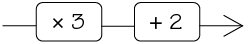(b) $9x-6$ (c)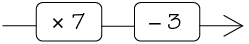$7x-3$
2. Which of the following pairs consist of like terms? Explain.

A.$3y; -7y$

B. $14e^2; 5e$

C $3y^2z; 17y^2z$

D. $-bcd; 5bd$

3. Write the following in the 'normal' algebraic way:
1. $c2 + d3$
2. $7 \times d \times e \times f$
4. Consider the expression $12x^2 - 5x + 3$.
1. What is the number 12 called?
2. Write down the coefficient of $x$.
3. What name is given to the number 3?
5. Explain why the terms $5pqr$ and $-10prq$ and $15qrp$ are like terms.
6. If $y =7$, what is the value of each of the following?
1. $y+8$
2. $9y$
3. $7 -y$
7. Simplify the following expressions:
1. $18c + 12d + 5c - 7c$
2. $3def + 4 + 7def - 6$
8. Evaluate the following expressions for $y = 3; z =-1$:
1. $2y^2 + 3z$
2. ($2y)^2 + 3z$
9. Write each algebraic expression in the simplest form.
1. $5y + 15y$
2. $5c + 6c - 3c + 2c$
3. $4b + 3 + 16b - 5$
4. $7m + 3n + 2 - 6m$
5. $5h^2 + 17 - 2h^2 + 3$
6. $7e^2f + 3ef + 2 + 4ef$
10. Evaluate the following expressions:
1. $3y+ 3y + 3y + 3y + 3y + 3y$ for $y = 18$
2. $13y + 14 - 3y + 6$ for $y = 200$
3. $20 - y^2 + 101y^2 + 80$ for $y = 1$
4. $12y^2 + 3yz + 18y^2 + 2yz$ for (\9y=3\) and $z = 2$

# Algebraic equations

In this chapter you will learn to find numbers that make certain statements true. A statement about an unknown number is called an equation. When we work to find out which number will make the equation true, we say we solve the equation. The number that makes the equation true is called the solution of the equation.

## Setting up equations

An equation is a mathematical sentence that is true for some numbers but false for other numbers. The following are examples of equations:

$x + 3 = 11 \text{ and } 2^x = 8$

$x + 3 = 11$ is true if $x =8$, but false if $x =3$.

When we look for a number or numbers that make an equation true we say that we are solving the equation. For example , $x = 4$ is the solution of $2x + 8$ because it makes $2x +8$true. (Check: $2 \times 4 = 8$

### Looking for numbers to make statements true

1. $x - 3 = 0$, if $x = -3$
2. $x^3 = 8$, if $x = -2$
3. $3x = -6$, if $x = -3$
4. $3x = 1$, if $x = 1$
5. $6x + 5 = 47$, if $x = 7$
2. Find the original number. Show your reasoning.
1. A number multiplied by 10 is 80.
3. Divide a number by 5 and the answer is 4.
4. Multiply a number by 4 and the answer is 20.
5. Twice a number is 100.
6. A number raised to the power 5 is 32.
7. A number raised to the power 4 is -81.
8. Fifteen times a number is 90.
9. 93 added to a number is -3.
10. Half a number is 15.
3. Write the equations below in words using "a number" in place of the letter symbol $x$. Then write what you think "the number" is in each case.

Example: $4 +x = 23$. Four plus a number equals twenty-three. The number is 19.

1. $8x = 72$
2. $\frac{2x}{5} = 2$

3. $2x+ 5 = 21$

4. $12 + 9x = 30$

5. $30 - 2x = 40$

6. $5x + 4 = 3x+ 10$

## Solving equations by inspection

### The answer is in plain sight

1. Seven equations are given below the table. Use the table to find out for which of the given values of $x$ it will be true that the left-hand side of the equation is equal to the right-hand side.

You can read the solutions of an equation from a table.

 $x$ -3 -2 -1 0 1 2 3 4 $2x + 3$ -3 -1 1 3 5 7 9 11 $x + 4$ 1 2 3 4 5 6 7 8 $9 -x$ 12 11 10 9 8 7 6 5 $3x -2$ -11 -8 -5 -2 1 4 7 10 $10x -7$ -37 -27 -17 -7 3 13 23 33 $5x +3$ -12 -7 -2 3 8 13 18 23 $10 - 3x$ 19 16 13 10 7 4 1 -2
1. $2x + 3 = 5x + 3$
2. $5x + 3 = 9 - x$
3. $2x + 3 = x+4$
4. $10x -7 = 5x + 3$
5. $3x -2 = x+4$
6. $9 -x = 2x + 3$
7. $10 -3x = 3x - 2$

Two or more equations can have the same solution. For example, $5x = 10$ and $x + 2 = 4$ have the same solution; $x = 2$ is the solution for both equations.

Two equations are called equivalent if they have the same solution.

1. Which of the equations in question 1 have the same solutions? Explain.
2. Complete the table below. Then answer the questions that follow.

You can also do a search by narrowing down the possible solution to an equation.

 $x$ 0 5 10 15 20 25 30 35 40 $2x + 3$ $3x -10$
1. Can you find a solution for $2x + 3 = 3x - 10$ in the table?
2. What happens to the values of $2x + 3$ and $3x - 10$ as $x$ increases? Do they become bigger or smaller?
3. Is there a point where the value of $3x - 10$ becomes bigger or smaller than the value of $2x + 3$ as the value of $x$ increases? If so, between which $x$-values does this happen?

This point where the two expressions are equal is called the break-even point.

4. Now that you narrowed down where the possible solution can be, try other possible values for $x$ until you find out for what value of $x$ the statement $2x + 3 = 3x - 10$ is true.

"Searching" for the solution of an equation by using tables or by narrowing down to the possible solution is called solution by inspection.

## More examples

### Looking for and checking solutions

1. What is the solution for the equations below?
1. $x - 3 = 4$
2. $x + 2 = 9$
3. $3x = 21$
4. $3x + 1 = 22$

When a certain number is the solution of an equation we say that the number satisfies the equation. For example, $x=4$ satisfies the equation $3x=12$ because $3 \times 4 = 12$.

1. Choose the number in brackets that satisfies the equation. Explain your choice.
1. $12x = 84$ {5; 7; 10; 12}
2. $\frac{84}{x} = 12$ {-7; 0; 7; 10}

3. $48 = 8k + 8$ {-5; 0; 5; 10}

4. $19 - 8m = 3$ {-2; -1; 0; 1; 2}

5. $20 = 6y - 4$ {3; 4; 5; 6}

6. $x^3 = -64$ {-8; -4; 4; 8}

7. $5^x =125$ {-3; -1; 1; 3}

8. $2^x = 8$ {1; 2; 3; 4}

9. $x^2 = 9$ {1; 2; 3; 4}

1. $m + 8 = 100$
2. $80 = x + 60$
3. $26 - k = 0$
4. $105 \times y = 0$
5. $k \times 10 = 10$
6. $5x = 100$
7. $\frac{15}{t} = 5$
8. $3 = \frac{t}{5}$
1. $12x + 14 = 50$
2. $100 = 15m + 25$
3. $\frac{100}{x} =20$
4. $7m + 5 = 40$
5. $2x + 8 = 10$
6. $3x + 10 = 31$
7. $-1 + 2x = -11$
8. $2 + \frac{x}{7} = 5$
9. $100 = 64 + 9x$
10. $\frac{2x}{6}= 4$

# Term revision and assessment

## Revision

Show all your steps of working.

### Whole numbers

1. Write both 300 and 160 as products of prime factors.
2. Determine the HCF and LCM of 300 and 160.
1. Tommy, Thami and Timmy are given birthday money by their grandmother in the ratio of their ages. They are turning 11, 13 and 16 years old, respectively. If the total amount of money given to all three boys is R1 000, how much money does Thami get on his birthday?
2. Tshepo and his family are driving to the coast on holiday. The distance is 1 200 km and they must reach their destination in 12 hours. After 5 hours, they have travelled 575 km. Then one of their tyres bursts. It takes 45 minutes to get the spare wheel on, before they can drive again. At what average speed must they drive the remainder of the journey to reach their destination on time?
3. The number of teachers at a school has increased in the ratio 5 : 6. If there used to be 25 teachers at the school, how many teachers are there now?
4. ABC for Life needs to have their annual statements audited. They are quoted R8 500 + 14% VAT by Audits Inc. How much will ABC for Life have to pay Audits Inc. in total?
5. Reshmi invests R35 000 for three years at an interest rate of 8,2% per annum. Determine how much money will be in her account at the end of the investment period.
6. Lesebo wants to buy a lounge suite that costs R7 999 cash. He does not have enough money and so decides to buy it on hire purchase. The store requires a 15% deposit up front, and 18 monthly instalments of R445.
1. Calculate the deposit that Lesebo must pay.
2. How much extra does Lesebo pay because he buys the lounge suite on hire purchase, rather than in cash?
7. Consider the following exchange rates table:
 South African Rand 1.00 ZAR inv. 1.00 ZAR Euro 0.075370 13.267807 US Dollar 0.098243 10.178807 British Pound 0.064602 15.479409 Indian Rupee 5.558584 0.179902 Australian Dollar 0.102281 9.776984 Canadian Dollar 0.101583 9.844200 Emirati Dirham 0.360838 2.771327 Swiss Franc 0.093651 10.677960 Chinese Yuan Renminbi 0.603065 1.658195 Malaysian Ringgit 0.303523 3.294646
1. Write down the amount in rand that needs to be exchanged to get 1 Swiss franc. Give your answer to the nearest cent.
2. Write down the only currency for which an exchange of R100 will give you more than 100 units of that currency.
3. Ntsako is travelling to Dubai and converts R10 000 into Emirati dirhams. How many dirhams does Ntsako receive (assume no commission)?

### Integers

Don't use a calculator for any of the questions in this section.

1. Write a number in each box to make the calculations correct.
1. ☐ + ☐ = -11
2. ☐ - ☐ = -11
2. Fill <, > or = into each block to show the relationships.
1. -23 ☐ 20
2. -345 ☐ -350
3. $4 - 3$ ☐ $3 - 4$
4. $5 - 7$ ☐ $-(7 - 5)$
5. $-9 \times 2$ ☐ $-9 \times -2$
6. $-4 \times 5$ ☐ $4 \times -5$
7. $-10 \div 5 ☐ -10 \div -2$
8. $-15 \times -15$ ☐ 224
3. Follow the pattern to complete the number sequences.
1. 8; 5; 2;☐
2. 2; -4; 8;☐
3. -289; -293; -297;☐
4. Look at the number lines. In each case, the missing number is halfway between the other two numbers. Fill in the correct values in the boxes.
1.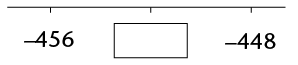2.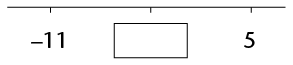5. Calculate the following:
1. $-5 - 7$
2. $7 - 10$
3. $8-(-9)$
4. $(-5)(-2)(-4)$
5. $5 + 4 \times 2$
6. $\frac{(\sqrt{4})(-2)^2}{-4}$
7. $\frac{-(-3)^3\sqrt{125}}{(-9)(3)}$
8. $\frac{\sqrt{-64}}{-3-1}$
1. Write down two numbers that multiply to give -15. (One of the numbers must be positive and the other negative.)
2. Write down two numbers that add to 15. One of the numbers must be positive and the other negative.
6. At 5 a.m., the temperature in Kimberley was -3 °C. At 1 p.m., it was 17 °C. By how many degrees had the temperature risen?
7. A submarine is 220 m below the surface of the sea. It travels 75 m upwards. How far below the surface is it now?

### Exponents

You should not use a calculator for any of the questions in this section.

1. Write down the value of the following:
1. $(-3)^3$
2. $-5^2$
3. $(-1)^{200}$
4. $(10^2)^2$
2. Write the following numbers in scientific notation:
1. 200 000
2. 12,345
3. Write the following numbers in ordinary notation:
1. $1,3 \times 10^2$
2. $7,01 \times 10^7$
4. Which of the following numbers is bigger: $5,23 \times 10^{10}$ or $2 ,9 \times 10^{11}$?
5. Simplify the following:
1. $2^7 \times 2^3$
2. $2x^3 \times 4x^4$
3. $(-8y^6) \div (4y^3)$
4. $(3x^8)^3$
5. $(2x^5)(0.5x^{-5})$
6. $(-3a^2b^3c)(-4abc^2)^2$
7. $\frac{(2xy^2z^3)(-5y^2z)^2}{20xy^8z^4}$
6. Write down the values of each of the following:
1. $(0,6)^2$
2. $(0,2)^3$
3. $(\frac{1}{2})^5$
4. $\sqrt{\frac{1}{4}}$
5. $4\sqrt{\frac{9}{64}}$
6. $\sqrt{0.001}$

### Numeric and geometric patterns

1. For each of the following sequences, write the rule for the relationship between each term and the following term in words. Then use the rule to write the next three terms in the sequence.
1. $12; 7; 2;\text{______};\text{______};\text{______}$
2. $-2; -6; -18;\text{______};\text{______};\text{______}$
3. $100; -50; 25;\text{______};\text{______};\text{______}$
4. $3; 4; 7; 11;\text{______};\text{______};\text{______}$
2. In this question, you are given the rule by which each term of the sequence can be found. In all cases, $n$ is the position of the term.

Determine the first three terms of each of the sequences. (Hint: Substitute $n = 1$ to find the value of the first term.)

1. $n \times 4$
2. $n\times 5 - 12$
3. $2 \times n^2$
4. $3n \div 3 \times -2$
3. Write down the rule by which each term of the sequence can be found (in a similar format to those given in question 2, where n is the position of the term).
1. $2; 4; 6; ...$
2. $-7; -3; 1; ...$
3. $2; 4; 8; ...$
4. $9; 16; 23; ...$
4. Use the rules you have found in question 3 to find the value of the 20th term of the sequences in questions 3(a) and 3(b).

5. Find the relationship between the position of the term in the sequence and the value of the term, and use it to fill in the missing values in the tables.
1.  Position in sequence 1 2 3 4 25 Value of the term -8 -11 -14
2.  Position in sequence 1 2 3 Value of the term 1 3 9 243 19 683
6. The image below shows a series of patterns created by matches.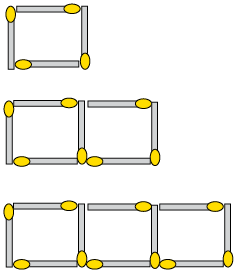1. Write in words the rule that describes the number of matches needed for each new pattern.
2. Use the rule to determine the missing values in the table below, and fill them in.
 Number of the pattern 1 2 3 4 20 Number of matches needed 4 7 151

### Functions and relationships

1. Fill in the missing input values, output values or rule in these flow diagrams. Note that $p$ and $t$ are integers.
1.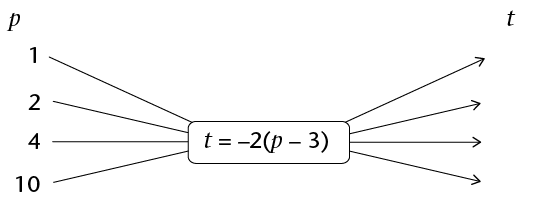2.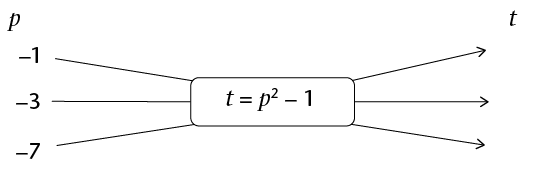3.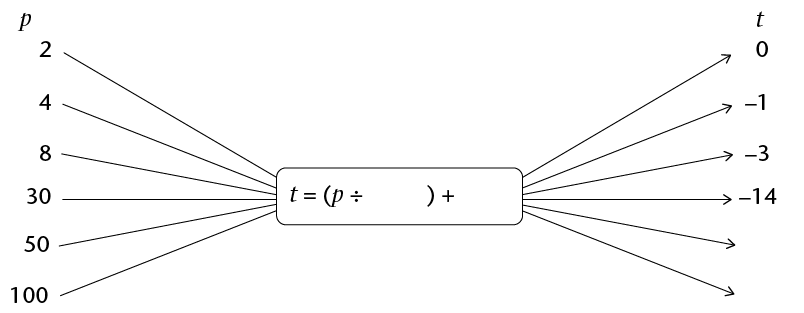2. Consider the values in the following table. The rule for finding $y$ is: divide $x$ by -2 and subtract 4. Use the rule to determine the missing values in the table, and write them in.
 x -2 0 2 5 y -1 -3 -4 48

3. Consider the values in the following table:
 $x$ -2 -1 0 1 2 4 15 $y$ 1 3 5 7 9 61
1. Write in words the rule for finding the $y$-values in the table.
2. Use the rule to determine the missing values in the table, and write them in.

### Algebraic expressions

1. Look at this algebraic expression: $5x^3 - 9 + 4x - 3x^2$.
1. How many terms does this expression have?
2. What is the variable in this expression?
3. What is the coefficient of the $x^2$ term?
4. What is the constant in this expression?
5. Rewrite the expression so that the terms are in order of decreasing powers of $x$.
2. In this question, $x=6$ and $y = 17$. Complete the rules to show different ways to determine $y$ if $x$ is known. The first way is done for you:

Way 1: Multiply x by 2 and add 5. This can be written as $y = 2x + 5$

1. Way 2: Multiply $x$ by ______ and then subtract ______. This can be written as
2. Way 3: Divide $x$ by ______ and then add ______ . This can be written as
3. Way 4: Add ______ to $x$, and then multiply by ______ . This can be written as
3. Simplify:
1. $2x^2 + 3x^2$
2. $9xy -12xy$
3. $3y^2 - 4y + 3y - 2y^2$
4. $9m^3 + 9m^2 + 9m^3 - 3$
4. Calculate the value of the following expressions if $a = -2; b = 3; c = -1 \text{ and } d = 0:$
1. $abc$
2. $-a^2$
3. $(abc)^d$
4. $a + b- 2c$
5. $(a + b)^{10}$

### Algebraic equations

1. Write equations that represent the given information:
1. Nandi is $x$ years old. Shaba, who is $y$ years old, is three years older than Nandi.
2. The temperature at Colesberg during the day was $x$°C. But at night, the temperature dropped by 15 degrees to reach -2°C.
2. Solve the following equations for $x$:
1. $x + 5 = 2$
2. $7 - x = 9$
3. $3x - 1 = -10$
4. $2x^3 = -16$
5. $2^x = 16$
6. $2(3)^x = 6$
3. If $3n - 1 = 11$, what is the value of $4n$?
4. If $c = a + b$ and $a + b + c = 16$ , determine the value of $c$.
1. If $2a + 3 = b$, write down values for $a$ and $a$ that will make the equation true.
2. Write down a different pair of values to make the equation true.

## Assessment

In this section, the numbers in brackets at the end of a question indicate the number of marks the question is worth. Use this information to help you determine how much working is needed. The total number of marks allocated to the assessment is 60.

1. The profits of GetRich Inc. have decreased in the ratio 5 : 3 due to the recession in the country. If their profits used to be R1 250 000, how much are their profits now?
2. Which car has the better rate of petrol consumption: Ashley's car, which drove 520 km on 32â„“ of petrol, or Zaza's car, which drove 880 km on 55â„“ of petrol? Show all your working.
3. Hanyani took out a R25 000 loan from a lender that charges him 22% interest each year. How much will he owe in one year's time?
4. Consider the following exchange rates table:
 South African Rand 1.00 ZAR inv. 1.00 ZAR Indian Rupee 5.558584 0.179902 Australian Dollar 0.102281 9.776984 Canadian Dollar 0.101583 9.844200 Emirati Dirham 0.360838 2.771327 Chinese Yuan Renminbi 0.603065 1.658195 Malaysian Ringgit 0.303523 3.294646

Chen returns from a business trip to Malaysia with 2 500 ringgit in his wallet. If he changes this money into rand in South Africa, how much will he receive?

5. Fill <, > or = into the block to show the relationship between the number expressions:
1. $6 - 4$ ☐ $4 - 6$
2. $2 \times -3$ ☐ $-3^2$
6. Look at the number sequence below. Fill in the next term into the block.

$-5; 10; -20$;☐

7. Calculate the following:
1. $(-4)^2 - 20$
2. $\sqrt{-8} + 14 \ \div 2$
8. Julius Caesar was a Roman emperor who lived from 100 BC to 44 BC. How old was he when he died?
1. Write down two numbers that divide to give an answer of -8. One of the numbers must be positive, and the other negative.
2. Write down two numbers that subtract to give an answer of 8. One of the numbers must be positive and the other negative.
9. Write the following number in scientific notation: 17 million.
10. Which of the following numbers is bigger: $3,47 \times 10^{21} \text{ or }7,99 \times 10^{20}$?
11. Simplify the following, leaving all answers with positive exponents:
1. $3^7 \times 3^-2$
2. $(-12y^8) \div (-3y^2)$
3. $\frac{(3xy^2z^3)(-yz)^2}{15x^5y^4z^7}$
12. Write down the values of each of the following:
1. $(0.3)^3$
2. $8 \sqrt{\frac{25}{16}}$
13. Consider the following number sequence: $2; -8; 32; ...$
1. Write in words the rule by which each term of the sequence can be found.
2. Write the next three terms in this sequence.
14. The picture below shows a series of patterns created by matches.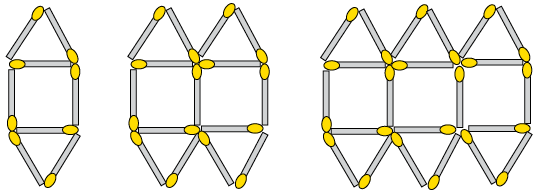1. Write a formula for the rule that describes the relationship between the number of matches and the position of the term in the sequence (pattern number). Let $n$ be the position of the term.
2. Use the rule to determine the values of $a$ to $c$ in the following table:
 Number of the pattern 1 2 3 4 15 c Number of matches needed 8 15 22 a b 148

15. Consider the values in the following table:
 x -2 -1 0 1 2 5 12 y -7 -4 -1 2 5 98
1. Write in words the rule for finding the $y$-values in the table.
2. Use the rule to determine the missing values in the table, and fill them in.
16. . Simplify:
1. $2z^2 - 3z^2$
2. $8y^2 - 6y + 4y - 7y^2$
17. Determine the value of $2a^2 - 10$ if $a = -2$.
18. If $c + 2d = 27$, give the value of the following:
1. $2c + 4d$
2. $\frac{c + 2d}{-9}$
3. $\sqrt{c + 2d}$
19. Solve the following for $x$: (5)
1. $-x - 11$
2. $2x -5 = - 11$
3. $4x^3 = 32$